The Australian Journal of Mathematical Analysis and Applications

 Home News Editors Volumes RGMIA Subscriptions Authors Contact

ISSN 1449-5910

Sorry death is imminent for file: ../public_html/searchroot/files/tex/v19n2/v19i2p4.tex
You searched for ando
Total of 449 results found in site

63: Paper Source PDF document

Paper's Title:

Convergence Speed of Some Random Implicit-Kirk-type Iterations for Contractive-type Random Operators

Author(s):

H. Akewe, K.S. Eke

Department of Mathematics,
Covenant University,
Canaanland, KM 10, Idiroko Road, P. M. B. 1023, Ota, Ogun State,
Nigeria.
E-mail: hudson.akewe@covenantuniversity.edu.ng, kanayo.eke@covenantuniversity.edu.ng

Abstract:

The main aim of this paper is to introduce a stochastic version of multistep type iterative scheme called a modified random implicit-Kirk multistep iterative scheme and prove strong convergence and stability results for a class of generalized contractive-type random operators. The rate of convergence of the random iterative schemes are also examined through an example. The results show that our new random implicit kirk multistep scheme perform better than other implicit iterative schemes in terms of convergence and thus have good potentials for further applications in equilibrium problems in computer science, physics and economics.

44: Paper Source PDF document

Paper's Title:

A Note On The Global Behavior Of A Nonlinear System of Difference Equations

Author(s):

Norman H. Josephy, Mihaela Predescu and Samuel W. Woolford

Department of Mathematical Sciences,
Bentley University,
Waltham, MA 02452,
U.S.A.

mpredescu@bentley.edu
njosephy@bentley.edu
swoolford@bentley.edu

Abstract:

This paper deals with the global asymptotic stability character of solutions of a discrete time deterministic model proposed by Wikan and Eide in Bulletin of Mathematical Biology, 66, 2004, 1685-1704. A stochastic extension of this model is proposed and discussed. Computer simulations suggest that the dynamics of the stochastic model includes a mixture of the dynamics observed in the deterministic model.

28: Paper Source PDF document

Paper's Title:

Essential Random Fixed Point Set of Random Operators

Author(s):

Ismat Beg

Centre for Advanced Studies in Mathematics,
Lahore University of Management Sciences (LUMS),
54792-Lahore, PAKISTAN. ibeg@lums.edu.pk
URL: http://web.lums.edu.pk/~ibeg

Abstract:

We obtain necessary and sufficient conditions for the existence of essential random fixed point of a random operator defined on a compact metric space. The structure of the set of essential random fixed points is also studied.

22: Paper Source PDF document

Paper's Title:

Maximal Inequalities for Multidimensionally Indexed Demimartingales and the Hájek-Rényi Inequality for Associated Random Variables

Author(s):

Tasos C. Christofides and Milto Hadjikyriakou

Department of Mathematics and Statistics
University of Cyprus
P.O.Box 20537, Nicosia 1678, Cyprus

tasos@ucy.ac.cy
miltwh@gmail.com

Abstract:

Demimartingales and demisubmartingales introduced by Newman and
Wright (1982) generalize the notion of martingales and
submartingales respectively. In this paper we define
multidimensionally indexed demimartingales and demisubmartingales
and prove a maximal inequality for this general class of random
variables. As a corollary we obtain a Hájek-Rényi inequality
for multidimensionally indexed associated random variables, the bound of which, when reduced to the case of single index, is sharper than the bounds already known in the literature.

20: Paper Source PDF document

Paper's Title:

Asymptotic Distribution of Products of Weighted Sums of Dependent Random Variables

Author(s):

Y. Miao and J. F. Li

College of Mathematics and Information Science,
Henan Normal University
Henan, China
yumiao728@yahoo.com.cn

College of Mathematics and Information Science,
Henan Normal University, 453007
Henan, China.
junfen_li@yahoo.com.cn

Abstract:

In this paper we establish the asymptotic distribution of products of weighted sums of dependent positive random variable, which extends the results of Rempała and Wesołowski (2002).

20: Paper Source PDF document

Paper's Title:

Bounds on the Jensen Gap, and Implications for Mean-Concentrated Distributions

Author(s):

Xiang Gao, Meera Sitharam, Adrian E. Roitberg

Department of Chemistry, and Department of Computer & Information Science & Engineering,
University of Florida,
Gainesville, FL 32611,
USA.
E-mail: qasdfgtyuiop@gmail.com

Abstract:

This paper gives upper and lower bounds on the gap in Jensen's inequality, i.e., the difference between the expected value of a function of a random variable and the value of the function at the expected value of the random variable. The bounds depend only on growth properties of the function and specific moments of the random variable. The bounds are particularly useful for distributions that are concentrated around the mean, a commonly occurring scenario such as the average of i.i.d. samples and in statistical mechanics.

17: Paper Source PDF document

Paper's Title:

Approximation of an AQCQ-Functional Equation and its Applications

Author(s):

Choonkil Park and Jung Rye Lee

Department of Mathematics,
Research Institute for Natural Sciences,
Hanyang University, Seoul 133-791,
Korea;

Department of Mathematics,
Daejin University,
Kyeonggi 487-711,
Korea

baak@hanyang.ac.kr
jrlee@daejin.ac.kr

Abstract:

This paper is a survey on the generalized Hyers-Ulam stability of an AQCQ-functional equation in several spaces. Its content is divided into the following sections:

1. Introduction and preliminaries.

2. Generalized Hyers-Ulam stability of an AQCQ-functional equation in Banach spaces: direct method.

3. Generalized Hyers-Ulam stability of an AQCQ-functional equation in Banach spaces: fixed point method.

4. Generalized Hyers-Ulam stability of an AQCQ-functional equation in random Banach spaces: direct method.

5. Generalized Hyers-Ulam stability of an AQCQ-functional equation in random Banach spaces: fixed point method.

6. Generalized Hyers-Ulam stability of an AQCQ-functional equation in non-Archi-medean Banach spaces: direct method.

7. Generalized Hyers-Ulam stability of an AQCQ-functional equation in non-Archi-medean Banach spaces: fixed point method.

15: Paper Source PDF document

Paper's Title:

Inequalities for Discrete F-Divergence Measures: A Survey of Recent Results

Author(s):

Sever S. Dragomir1,2

1Mathematics, School of Engineering & Science
Victoria University, PO Box 14428
Melbourne City, MC 8001,
Australia
E-mail: sever.dragomir@vu.edu.au

2DST-NRF Centre of Excellence in the Mathematical and Statistical Sciences,
School of Computer Science & Applied Mathematics,
University of the Witwatersrand,
Private Bag 3, Johannesburg 2050,
South Africa
URL: http://rgmia.org/dragomir

Abstract:

In this paper we survey some recent results obtained by the author in providing various bounds for the celebrated f-divergence measure for various classes of functions f. Several techniques including inequalities of Jensen and Slater types for convex functions are employed. Bounds in terms of Kullback-Leibler Distance, Hellinger Discrimination and Varation distance are provided. Approximations of the f-divergence measure by the use of the celebrated Ostrowski and Trapezoid inequalities are obtained. More accurate approximation formulae that make use of Taylor's expansion with integral remainder are also surveyed. A comprehensive list of recent papers by several authors related this important concept in information theory is also included as an appendix to the main text.

15: Paper Source PDF document

Paper's Title:

A Comparison Between Two Different Stochastic Epidemic Models with Respect to the Entropy

Author(s):

Department of Mathematics,
University of Sussex,
Brighton BN1 9QH,
UK.
E-mail: f.fatehi@sussex.ac.uk
URL: http://www.sussex.ac.uk/profiles/361251

Department of Pure Mathematics, Faculty of Mathematics and Computer,
Shahid Bahonar University of Kerman,
Kerman 76169-14111,
Iran.

Abstract:

In this paper at first a brief history of mathematical models is presented with the aim to clarify the reliability of stochastic models over deterministic models. Next, the necessary background about random variables and stochastic processes, especially Markov chains and the entropy are introduced. After that, entropy of SIR stochastic models is computed and it is proven that an epidemic will disappear after a long time. Entropy of a stochastic mathematical model determines the average uncertainty about the outcome of that random experiment. At the end, we introduce a chain binomial epidemic model and compute its entropy, which is then compared with the DTMC SIR epidemic model to show which one is nearer to reality.

13: Paper Source PDF document

Paper's Title:

Some Properties of the Marshall-Olkin and Generalized Cuadras-Augé Families of Copulas

Author(s):

Edward Dobrowolski and Pranesh Kumar

Department of Mathematics and Statistics
University of Northern British Columbia
Prince George, BC,

E-mail: Pranesh.Kumar@unbc.ca

Abstract:

We investigate some properties of the families of two parameter Marshall-Olkin and Generalized Cuadras-Augé copulas. Some new results are proved for copula parameters, dependence measure and mutual information. A numerical application is discussed.

12: Paper Source PDF document

Paper's Title:

On the Convergence in Law of Iterates of Random-Valued Functions

Author(s):

Karol Baron

Uniwersytet Śląski, Instytut Matematyki
Bankowa 14, PL--40--007 Katowice,
Poland
baron@us.edu.pl

Abstract:

Given a probability space (Ω, A, P) a separable and complete metric space X with the σ-algebra B of all its Borel subsets and a B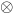A -measurable f : X * Ω → X we consider its iterates fn, n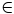N, defined on X * ΩN by f1(x,ω) = f(x,ω1)  and fn+1(x,ω)=f(fn(x,ω),ωn+1), provide a simple criterion for the convergence in law of fn(x,·)) nN, to a random variable independent of xX , and apply this criterion to linear functional equations in a single variable.

11: Paper Source PDF document

Paper's Title:

A note on Inequalities due to Martins, Bennett and Alzer

Author(s):

József Sándor

Babeş-Bolyai University of Cluj, Department of Mathematics and Computer Sciences
Kogălniceanu Nr.1, Cluj-Napoca,
Romania.
jjsandor@hotmail.com
jsandor@member.ams.org

Abstract:

A short history of certain inequalities by Martins, Bennett as well as Alzer, is provided. It is shown that, the inequality of Alzer for negative powers , or Martin's reverse inequality  are due in fact to Alzer . Some related results, as well as a conjecture, are stated.

10: Paper Source PDF document

Paper's Title:

1-type Pseudo-Chebyshev Subspaces in Generalized 2-normed Spaces

Author(s):

Sh. Rezapour

Department of Mathematics, Azarbaijan University of Tarbiat Moallem,
Azarshahr, Tabriz,
Iran
sh.rezapour@azaruniv.edu

Abstract:

We construct a generalized 2-normed space from every normed space. We introduce 1-type pseudo-Chebyshev subspaces in generalized 2-normed spaces and give some results in this field.

7: Paper Source PDF document

Paper's Title:

Weak solutions of non coercive stochastic Navier-Stokes equations in R2

Author(s):

Wilhelm Stannat and Satoshi Yokoyama

Technische Universität Berlin,
Strasse des 17. Juni 136, 10623 Berlin,
Germany.

The University of Tokyo,
Komaba, Tokyo 153-8914,
Japan.

E-mail: stannat@math.tu-berlin.de

E-mail: satoshi2@ms.u-tokyo.ac.jp

Abstract:

We prove existence of weak solutions of stochastic Navier-Stokes equations in R2 which do not satisfy the coercivity condition. The equations are formally derived from the critical point of some variational problem defined on the space of volume preserving diffeomorphisms in R2. Since the domain of our equation is unbounded, it is more difficult to get tightness of approximating sequences of solutions in comparison with the case of a bounded domain. Our approach is based on uniform a priori estimates on the enstrophy of weak solutions of the stochastic 2D-Navier-Stokes equations with periodic boundary conditions, where the periodicity is growing to infinity combined with a suitable spatial cutoff-technique.

7: Paper Source PDF document

Paper's Title:

Antiderivatives and Integrals Involving Incomplete Beta Functions with Applications

Author(s):

Mathematics Department,
Yarmouk University,
Irbid 21163,
Jordan.
E-mail: rami_thenat@yu.edu.jo

Faculty of Engineering,
Higher Colleges of Technology,
Ras Alkhaimah,
UAE.

Abstract:

In this paper, we prove that incomplete beta functions are antiderivatives of several products and powers of trigonometric functions, we give formulas for antiderivatives for products and powers of trigonometric functions in term of incomplete beta functions, and we evaluate integrals involving trigonometric functions using incomplete beta functions. Also, we extend some properties of the beta functions to the incomplete beta functions. As an application for the above results, we find the moments for certain probability distributions.

6: Paper Source PDF document

Paper's Title:

Bartle Integration in Lie Algebras

Author(s):

Andreas Boukas and Philip Feinsilver

Centro Vito Volterra,
Universita di Roma Tor Vergata,
via Columbia 2, 00133 Roma,
Italy.

Department of Mathematics,
Southern Illinois University,
Carbondale, Illinois 62901,
USA.

E-mail: andreasboukas@yahoo.com
E-mail:  pfeinsil@math.siu.edu

Abstract:

Using Bartle's bilinear vector integral we define stochastic integrals of bounded operator valued functions with respect to Stieltjes measures associated with the generators of the Heisenberg and Finite Difference Lie algebras. Our definition also covers the Square of White Noise and sl/2 Lie algebras.

6: Paper Source PDF document

Paper's Title:

Ostrowski Type Inequalities for Lebesgue Integral: a Survey of Recent Results

Author(s):

Sever S. Dragomir1,2

1Mathematics, School of Engineering & Science
Victoria University, PO Box 14428
Melbourne City, MC 8001,
Australia
E-mail: sever.dragomir@vu.edu.au

2DST-NRF Centre of Excellence in the Mathematical and Statistical Sciences,
School of Computer Science & Applied Mathematics,
University of the Witwatersrand,
Private Bag 3, Johannesburg 2050,
South Africa
URL: http://rgmia.org/dragomir

Abstract:

The main aim of this survey is to present recent results concerning Ostrowski type inequalities for the Lebesgue integral of various classes of complex and real-valued functions. The survey is intended for use by both researchers in various fields of Classical and Modern Analysis and Mathematical Inequalities and their Applications, domains which have grown exponentially in the last decade, as well as by postgraduate students and scientists applying inequalities in their specific areas.

6: Paper Source PDF document

Paper's Title:

Fractional Integral Inequalities of Hermite-Hadamard Type for P-convex and Quasi-Convex Stochastic Process

Author(s):

Oualid Rholam, Mohammed Barmaki and Driss Gretet

National School of Applied Sciences (ENSA),
University Ibn Tofail,
B.P 242 Kenitra 14000,
phone number : +212606257757,
Morocco.
E-mail: oualid.rholam@uit.ac.ma

Science Faculty Ben M'sik,
University Hassan II,
B.P 7955 Av Driss El Harti Sidi Othmane 20700,
phone number : +212 5 22 70 46 71 ,
Morocco.
E-mail:  mohammed.barmaki@uit.ac.ma

National School of Applied Sciences (ENSA),
University Ibn Tofail,
B.P 242 Kenitra 14000,
phone number : +212661403557,
Morocco.
E-mail: driss.gretete@uit.ac.ma

Abstract:

In this paper we consider the class of P-convex and Quasi-convex stochastic processes on witch we apply a general class of generalized fractional integral operator in order to establish new integral inequalities of Hermite-Hadammard type. then we obtain some results for well known types of fractional integrals. Results obtained in this paper may be starting point as well as a useful source of inspiration for further research in convex analysis.

5: Paper Source PDF document

Paper's Title:

Some Homogeneous Cyclic Inequalities of Three Variables of Degree Three and Four

Author(s):

TETSUYA ANDO

Department of Mathematics and Informatics,
Chiba University, Chiba 263-8522, JAPAN

ando@math.s.chiba-u.ac.jp

Abstract:

We shall show that the three variable cubic inequality
t2 (a3+b3+c3) + (t4-2t)(ab2+bc2+ca2)
≥ (2t3-1)(a2b+b2c+c2a) + (3t4-6t3+3t2-6t+3)abc

holds for non-negative a, b, c, and for any real number t.
We also show some similar three variable cyclic quartic inequalities.

5: Paper Source PDF document

Paper's Title:

On Reformations of 2--Hilbert Spaces

Author(s):

M. Eshaghi Gordji, A. Divandari, M. R. Safi and Y. J. Cho

Department of Mathematics, Semnan University,
P.O. Box 35195--363, Semnan,
Iran

Department of Mathematics, Semnan University,
Iran

Divandari@sun.Semnan.ac.ir

Department of Mathematics, Semnan University,
Iran

Department of Mathematics Education and the RINS,
Gyeongsang National University
Chinju 660-701,
Korea

yjcho@gnu.ac.kr

Abstract:

In this paper, first, we introduce the new concept of (complex) 2--Hilbert spaces, that is, we define the concept of 2--inner product spaces with a complex valued 2--inner product by using the 2--norm. Next, we prove some theorems on Schwartz's inequality, the polarization identity, the parallelogram laws and related important properties. Finally, we give some open problems related to 2--Hilbert spaces.

4: Paper Source PDF document

Paper's Title:

A Wallis Type Inequality and a Double Inequality for Probability Integral

Author(s):

Jian Cao, Da-Wei Niu and Feng Qi

School of Mathematics and Informatics,
Henan Polytechnic University, Jiaozuo City,
Henan Province, 454010,
China
21caojian@163.com

School of Mathematics and Informatics,
Henan Polytechnic University, Jiaozuo City,
Henan Province, 454010,
China
nnddww@tom.com

Research Institute of Mathematical Inequality Theory,
Henan Polytechnic University, Jiaozuo City,
Henan Province, 454010,
China
qifeng@hpu.edu.cn
fengqi618@member.ams.org
qifeng618@hotmail.com
qifeng618@msn.com
qifeng618@qq.com
URL: http://rgmia.vu.edu.au/qi.html

Abstract:

In this short note, a Wallis type inequality with the best upper and lower bounds is established. As an application, a double inequality for the probability integral is found.

4: Paper Source PDF document

Paper's Title:

Existence of solutions for Neutral Stochastic Functional Differential Systems with Infinite Delay in Abstract Space

Author(s):

P. Balasubramaniam, A. V. A. Kumar and S. K. Ntouyas

Department of Mathematics, Gandhigram Rural Institute,
Deemed University, Gandhigram - 624 302, Tamil Nadu, India.
pbalgri@rediffmail.com

Department of Mathematics, Gandhigram Rural Institute,
Deemed University, Gandhigram - 624 302, Tamil Nadu, India.
nnddww@tom.com

Department of Mathematics, University of Ioannina,
451 10 Ioannina,
Greece.
sntouyas@cc.uoi.gr
URL: http://www.math.uoi.gr/~sntouyas

Abstract:

In this paper we prove existence results for semilinear stochastic neutral functional differential systems with unbounded delay in abstract space. Our theory makes use of analytic semigroups and fractional power of closed operators and Sadovskii fixed point theorem.

4: Paper Source PDF document

Paper's Title:

A Double Inequality for Divided Differences and Some Identities of the Psi and Polygamma Functions

Author(s):

B. N. Guo and F. Qi

School of Mathematics and Informatics, Henan Polytechnic University, Jiaozuo City, Henan Province, 454010, China
bai.ni.guo@gmail.com, bai.ni.guo@hotmail.com

School of Research Institute of Mathematical Inequality Theory, Henan Polytechnic University, Jiaozuo City, Henan Province, 454010, China
qifeng618@gmail.com, qifeng618@hotmail.com, qifeng618@qq.com
URL:http://qifeng618.spaces.live.com

Abstract:

In this short note, from the logarithmically completely monotonic property of the function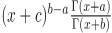, a double inequality for the divided differences and some identities of the psi and polygamma functions are presented.

3: Paper Source PDF document

Paper's Title:

On Stable Numerical Differentiation

Author(s):

N. S. Hoang and A. G. Ramm

Mathematics Department, Kansas State University
Manhattan, KS 66506-2602,
U. S. A.
nguyenhs@math.ksu.edu
ramm@math.ksu.edu
URL:http://math.ksu.edu/~ramm

Abstract:

Based on a regularized Volterra equation, two different approaches for numerical differentiation are considered. The first approach consists of solving a regularized Volterra equation while the second approach is based on solving a disretized version of the regularized Volterra equation. Numerical experiments show that these methods are efficient and compete favorably with the variational regularization method for stable calculating the derivatives of noisy functions.

3: Paper Source PDF document

Paper's Title:

A Study of the Effect of Density Dependence in a Matrix Population Model

Author(s):

N. Carter and M. Predescu

Department of Mathematical Sciences,
Bentley University,
Waltham, MA 02452,
U.S.A.
ncarter@bentley.edu
mpredescu@bentley.edu

Abstract:

We study the behavior of solutions of a three dimensional discrete time nonlinear matrix population model. We prove results concerning the existence of equilibrium points, boundedness, permanence of solutions, and global stability in special cases of interest. Moreover, numerical simulations are used to compare the dynamics of two main forms of the density dependence function (rational and exponential).

3: Paper Source PDF document

Paper's Title:

On the Fock Representation of the Central Extensions of the Heisenberg Algebra

Author(s):

L. Accardi and A. Boukas

Centro Vito Volterra, Universitŕ di Roma Tor Vergata,
via Columbia 2, 00133 Roma,
Italy
accardi@volterra.mat.uniroma2.it
URL: http://volterra.mat.uniroma2.it

Department of Mathematics,
American College of Greece,
Greece
andreasboukas@acg.edu

Abstract:

We examine the possibility of a direct Fock representation of the recently obtained non-trivial central extensions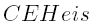of the Heisenberg algebra, generated by elements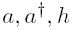and E satisfying the commutation relations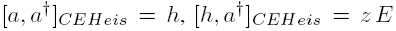,  and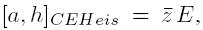, where a and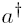are dual, h is self-adjoint, E is the non-zero self-adjoint central element and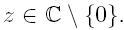We define the exponential vectors associated with theFock space, we compute their Leibniz function (inner product), we describe the action of a,and h on the exponential vectors and we compute the moment generating and characteristic functions of the classical random variable corresponding to the self-adjoint operator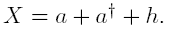3: Paper Source PDF document

Paper's Title:

Some Functional Inequalities for the Geometric Operator Mean

Author(s):

Mustapha Raissouli

Taibah University, Faculty of Sciences, Department of Mathematics,
Al Madinah Al Munawwarah, P.O.Box 30097,
Kingdom of Saudi Arabia.

raissouli_10@hotmail.com

Abstract:

In this paper, we give some new inequalities of functional type for the power geometric operator mean involving several arguments.

3: Paper Source PDF document

Paper's Title:

New Inequalities of Mill's Ratio and Application to The Inverse Q-function Approximation

Author(s):

Pingyi Fan

Department of Electronic Engineering,
Tsinghua University, Beijing,
China

fpy@tsinghua.edu.cn

Abstract:

In this paper, we investigate the Mill's ratio estimation problem and get two new inequalities. Compared to the well known results obtained by Gordon, they becomes tighter. Furthermore, we also discuss the inverse Q-function approximation problem and present some useful results on the inverse solution. Numerical results confirm the validness of our theoretical analysis. In addition, we also present a conjecture on the bounds of inverse solution on Q-function.

3: Paper Source PDF document

Paper's Title:

Some New Generalizations of Jensen's Inequality with Related Results and Applications

Author(s):

Steven G. From

Department of Mathematics

E-mail: sfrom@unomaha.edu

Abstract:

In this paper, some new generalizations of Jensen's inequality are presented. In particular, upper and lower bounds for the Jensen gap are given and compared analytically and numerically to previously published bounds for both the discrete and continuous Jensen's inequality cases. The new bounds compare favorably to previously proposed bounds. A new method based on a series of locally linear interpolations is given and is the basis for most of the bounds given in this paper. The wide applicability of this method will be demonstrated. As by-products of this method, we shall obtain some new Hermite-Hadamard inequalities for functions which are 3-convex or 3-concave. The new method works to obtain bounds for the Jensen gap for non-convex functions as well, provided one or two derivatives of the nonlinear function are continuous. The mean residual life function of applied probability and reliability theory plays a prominent role in construction of bounds for the Jensen gap. We also present an exact integral representation for the Jensen gap in the continuous case. We briefly discuss some inequalities for other types of convexity, such as convexity in the geometric mean, and briefly discuss applications to reliability theory.

3: Paper Source PDF document

Paper's Title:

On Singular Numbers of Hankel Matrices of Markov Functions

Author(s):

Vasily A. Prokhorov

Department of Mathematics and Statistics,
University of South Alabama,
Mobile, Alabama 36688-0002,
USA.
E-mail: prokhoro@southalabama.edu
URL: http://www.southalabama.edu/mathstat/people/prokhorov.shtml

Abstract:

Let E ⊂ (01,1) be a compact set and let μ be a positive Borel measure with support supp μ=E. Let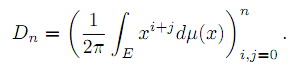In the case when E=[a,b]⊂ (-1,1) and μ satisfies the condition dμ/dx>0 a.e. on E, we investigate asymptotic behavior of singular numbers σkn,n of the Hankel matrix Dn, where kn/n→θ∈[0,1] as n→∞. Moreover, we obtain asymptotics of the Kolmogorov, Gelfand and linear k-widths, k=kn, of the unit ball An,2 of Pn∩L2(Γ) in the space L2(μ,E), where Γ={z:|z|=1} and Pn is the class of all polynomials of the degree at most n.

3: Paper Source PDF document

Paper's Title:

Existence of Solution of Differential and Riemann-Liouville Equation Via Fixed Point Approach in Complex Valued b-Metric Spaces

Author(s):

K. Afassinou, A. A. Mebawondu, H. A. Abass and O. K. Narain

Department of Science Access,
South Africa.
E-mail: komia@aims.ac.za

DST-NRF Centre of Excellence in Mathematical and Statistical Sciences (CoE-MaSS),
Johannesburg,
South Africa.
E-mail: dele@aims.ac.za

DST-NRF Centre of Excellence in Mathematical and Statistical Sciences (CoE-MaSS),
Johannesburg,
South Africa.
E-mail: hammedabass548@gmail.com

School of Mathematics, Statistics and Computer Science,
University of KwaZulu-Natal, Durban,
South Africa.
E-mail: naraino@ukzn.ac.za

Abstract:

In this paper, we establish some fixed point and common fixed point results for a new type of generalized contractive mapping using the notion of C-class function in the framework of complex valued b-metric spaces. As an application, we establish the existence and uniqueness of a solution for Riemann-Liouville integral and ordinary differential equation in the framework of a complete complex valued b-metric spaces. The obtained results generalize and improve some fixed point results in the literature.

3: Paper Source PDF document

Paper's Title:

Lozi Maps With Max Function and its Application

Author(s):

Abdellah Menasri

Higher National School of Forests,
Khenchela,
Algeria.
E-mail: abdellah.menasri70@gmail.com

Abstract:

In this paper, we study the Lozi map by replacing the piecewise linear term in the first equation by the function max (f(x,y);g(x,y)) such that f and g are two arbitrary functions in R2. This is a family model that allows us to study several new piecewise-smooth maps. We demonstrate that these models converge to a robust chaotic attractor and give some applications of these models in the real world.

2: Paper Source PDF document

Paper's Title:

On a Criteria for Strong Starlikeness

Author(s):

V. Ravichandran, M. Darus, and N. Seenivasagan

School Of Mathematical Sciences, Universiti Sains Malaysia,
11800 Usm Penang, Malaysia
vravi@cs.usm.my
URL: http://cs.usm.my/~vravi

School of Mathematical Sciences, Faculty of Sciences and Technology,
Ukm, Bangi 43600, Malaysia
maslina@pkrisc.cc.ukm.my

Sindhi College, 123, P. H. Road, Numbal,
Chennai 600 077 India
vasagan2000@yahoo.co.in

Abstract:

In this paper, we are concerned with finding sufficient condition for certain normalized analytic function f(z) defined on the open unit disk in the complex plane to be strongly starlike of order α. Also we have obtained similar results for certain functions defined by Ruscheweyh derivatives and Sălăgean derivatives. Further extension of these results are given for certain p-valent analytic functions defined through a linear operator.

2: Paper Source PDF document

Paper's Title:

A New Step Size Rule in Noor's Method for Solving General Variational Inequalities

Author(s):

Abdellah Bnouhachem

School of Management Science and Engineering, Nanjing University,
Nanjing, 210093
P.R. China.
babedallah@yahoo.com

Abstract:

In this paper, we propose a new step size rule in Noor's method for solving general variational inequalities. Under suitable conditions, we prove that the new method is globally convergent. Preliminary numerical experiments are included to illustrate the advantage and efficiency of the proposed method.

2: Paper Source PDF document

Paper's Title:

Long Correlations Applied to the Study of Agricultural Indices in Comparison with the S&P500 index

Author(s):

M. C. Mariani, J. Libbin, M.P. Beccar Varela,
V. Kumar Mani, C. Erickson, D.J. Valles Rosales

Department of Mathematical Sciences,
Science Hall 236, New Mexico State University,
Las Cruces, NM 88003-8001,
USA.
mmariani@nmsu.edu

Abstract:

Long-time correlations in agricultural indices are studied and their behavior is compared to the well-established S&P500 index. Hurst exponent and Detrended Fluctuation Analysis (DFA) techniques are used in this analysis. We detected long-correlations in the agricultural indices and briefly discussed some features specific in comparison to the S&P500 index.

2: Paper Source PDF document

Paper's Title:

Topological Aspects of Scalarization in Vector Optimization Problems.

Author(s):

Peter I. Kogut, Rosanna Manzo and Igor V. Nechay

Department of Differential Equations,
Dnipropetrovsk National University, Naukova STR.,
13, 49010 Dnipropetrovsk,
Ukraine

p.kogut@i.ua

Universitŕ di Salerno,
Dipartimento di Ingegneria dell'Informazione e Matematica Applicata,
Via Ponte don Melillo, 84084 Fisciano (SA),
Italy

manzo@diima.unisa.it

Department of Technical Cybernetics,
Dnipropetrovsk Technical University,
Acad. Lazarjan STR., 2, 49010 Dnipropetrovsk,
Ukraine

i.nechay@i.ua

Abstract:

In this paper, we study vector optimization problems in partially ordered Banach spaces. We suppose that the objective mapping possesses a weakened property of lower semicontinuity and make no assumptions on the interior of the ordering cone. We derive sufficient conditions for existence of efficient solutions of the above problems and discuss the role of topological properties of the objective space. We discuss the scalarization of vector optimization problems when the objective functions are vector-valued mappings with a weakened property of lower semicontinuity. We also prove the existence of the so-called generalized efficient solutions via the scalarization process. All principal notions and assertions are illustrated by numerous examples.

2: Paper Source PDF document

Paper's Title:

Shape Diagrams for 2D Compact Sets - Part III: Convexity Discrimination for Analytic and Discretized Simply Connected Sets.

Author(s):

S. Rivollier, J. Debayle and J.-C. Pinoli

Ecole Nationale Supérieure des Mines de Saint-Etienne,
CIS - LPMG, UMR CNRS 5148, 158 cours Fauriel,
42023 Saint-Etienne Cedex 2, France.

Abstract:

Shape diagrams are representations in the Euclidean plane introduced to study 3-dimensional and 2-dimensional compact convex sets. However, they can also been applied to more general compact sets than compact convex sets. A compact set is represented by a point within a shape diagram whose coordinates are morphometrical functionals defined as normalized ratios of geometrical functionals. Classically, the geometrical functionals are the area, the perimeter, the radii of the inscribed and circumscribed circles, and the minimum and maximum Feret diameters. They allow twenty-two shape diagrams to be built. Starting from these six classical geometrical functionals, a detailed comparative study has been performed in order to analyze the representation relevance and discrimination power of these twenty-two shape diagrams. The two first parts of this study are published in previous papers 8,9. They focus on analytic compact convex sets and analytic simply connected compact sets, respectively. The purpose of this paper is to present the third part, by focusing on the convexity discrimination for analytic and discretized simply connected compact sets..

2: Paper Source PDF document

Paper's Title:

On the Inequality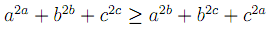Author(s):

A. Coronel and F. Huancas

Departamento de Ciencias Básicas,
Campus Fernando May, Chillán, Chile.

acoronel@roble.fdo-may.ubiobio.cl

Facultad de Ciencias Físicas y Matemáticas,
Universidad Nacional Pedro Ruiz Gallo, Juan XIII s/n,
Lambayeque, Perú

Abstract:

In this paper we give a complete proof offor all positive real numbers a, b and c. Furthermore, we present another way to prove the statement for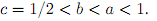2: Paper Source PDF document

Paper's Title:

Expected Utility with Subjective Events

Author(s):

Jacob Gyntelberg and Frank Hansen

Bank for International Settlements,
Basel,
Switzerland

jacob.gyntelberg@bis.org

Tohoku University, Institute for International Education,
Sendai,
Japan

frank.hansen@m.tohoku.ac.jp

Abstract:

We provide a new theory of expected utility with subjective events modeled by a lattice of projections. This approach allows us to capture the notion of a ``small world'' as a context dependent or local state space embedded into a subjective set of events, the ``grand world''. For each situation the decision makers' subjective ``small world'' reflects the events perceived to be relevant for the act under consideration. The subjective set of events need not be representable by a classical state space. Maintaining preference axioms similar in spirit to the classical axioms, we obtain an expected utility representation which is consistent across local state spaces and separates subjective probability and utility. An added benefit is that this alternative expected utility representation allows for an intuitive distinction between risk and uncertainty.

2: Paper Source PDF document

Paper's Title:

On Some Estimates for the Logarithmic Mean

Author(s):

Department of Information and Computer Engineering,
Kisarazu National College of Technology,
Kisarazu, Chiba 292-0041,
Japan.

Abstract:

We show some estimates for the logarithmic mean that are obtained from operator inequalities between the Barbour path and the Heinz means.

2: Paper Source PDF document

Paper's Title:

New Stochastic Calculus

Author(s):

Moawia Alghalith

Department of Economics,
University of the West Indies, St. Augustine,

E-mail: malghalith@gmail.com

Abstract:

We present new stochastic differential equations, that are more general and simpler than the existing Ito-based stochastic differential equations. As an example, we apply our approach to the investment (portfolio) model.

2: Paper Source PDF document

Paper's Title:

The Conservativeness of Girsanov Transformed for Symmetric Jump-diffusion Process

Author(s):

Mila Kurniawaty and Marjono

Department of Mathematics,
Universitas Brawijaya,
Malang,
Indonesia.
E-mail: mila_n12@ub.ac.id, marjono@ub.ac.id

Abstract:

We study about the Girsanov transformed for symmetric Markov processes with jumps associated with regular Dirichlet form. We prove the conservativeness of it by dividing the regular Dirichlet form  into the "small jump" part and the "big jump" part.

2: Paper Source PDF document

Paper's Title:

Solving Two Point Boundary Value Problems by Modified Sumudu Transform Homotopy Perturbation Method

Author(s):

Asem AL Nemrat and Zarita Zainuddin

School of Mathematical Sciences,
Universiti Sains Malaysia,
11800 Penang,
Malaysia.
E-mail: alnemrata@yahoo.com
zarita@usm.my

Abstract:

This paper considers a combined form of the Sumudu transform with the modified homotopy perturbation method (MHPM) to find approximate and analytical solutions for nonlinear two point boundary value problems. This method is called the modified Sumudu transform homotopy perturbation method (MSTHPM). The suggested technique avoids the round-off errors and finds the solution without any restrictive assumptions or discretization. We will introduce an appropriate initial approximation and furthermore, the residual error will be canceled in some points of the interval (RECP). Only a first order approximation of MSTHPM will be required, as compared to STHPM, which needs more iterations for the same cases of study. After comparing figures between approximate, MSTHPM, STHPM and numerical solutions, it is found through the solutions we have obtained that they are highly accurate, indicating that the MSTHPM is very effective, simple and can be used to solve other types of nonlinear boundary value problems (BVPs).

2: Paper Source PDF document

Paper's Title:

A new approach to the study of fixed point for simulation functions with application in G-metric spaces

Author(s):

Komi Afassinou and Ojen Kumar Narain

Department of Mathematical Sciences, University of Zululand,
South Africa.
E-mail: komia@aims.ac.za

School of Mathematics, Statistics and Computer Science,
University of KwaZulu-Natal, Durban,
South Africa.
E-mail: naraino@ukzn.ac.za

Abstract:

The purpose of this work is to generalize the fixed point results of Kumar et al.  by introducing the concept of (α,β)-Z-contraction mapping, Suzuki generalized (α,β)-Z-contraction mapping, (α,β)-admissible mapping and triangular (α,β)-admissible mapping in the frame work of G-metric spaces. Fixed point theorems for these class of mappings are established in the frame work of a complete G-metric spaces and we establish a generalization of the fixed point result of Kumar et al.  and a host of others in the literature. Finally, we apply our fixed point result to solve an integral equation.

2: Paper Source PDF document

Paper's Title:

Several Applications of a Local Non-convex Young-type Inequality

Author(s):

Loredana Ciurdariu, Sorin Lugojan

Department of Mathematics,
"Politehnica" University of Timisoara,
P-ta. Victoriei, No.2, 300006-Timisoara,
Romania.

E-mail: ltirtirau87@yahoo.com

Abstract:

A local version of the Young inequality for positive numbers is used in order to deduce some inequalities about determinants and norms for real quadratic matrices and norms of positive operators on complex Hilbert spaces.

2: Paper Source PDF document

Paper's Title:

Inequalities of Gamma Function Appearing in Generalizing Probability Sampling Design

Author(s):

Department of Mathematics,
Yarmouk University,
Irbid 21163,
Jordan.

Department of Statistics,
Yarmouk University,
Irbid 21163,
Jordan.

Abstract:

In this paper, we investigate the complete monotonicity of some functions involving gamma function. Using the monotonic properties of these functions, we derived some inequalities involving gamma and beta functions. Such inequalities can be used to generalize different probability distribution functions. Also, they can be used to generalize some statistical designs, e.g., the probability proportional to the size without replacement design.

2: Paper Source PDF document

Paper's Title:

On Admissible Mapping via Simulation Function

Author(s):

Department of Mathematics,
Faculty of Science and Technology,
Rambhai Barni Rajabhat University,
Chanthaburi 22000,
Thailand.
E-mail: anantachai.p@rbru.ac.th

Department of Mathematics and Computer Science
Faculty of Science and Technology
Rajamangala University of Technology Thanyaburi (RMUTT),
Thanyaburi, Pathumthani 12110,
Thailand.
E-mail: pakeeta_s@rmutt.ac.th

Abstract:

In this paper, we present some fixed point results in complete metric spaces by using generalized admissible mapping embedded in the simulation function. Its applications, Our results used to study the existence problem of nonlinear Hammerstein integral equations.

2: Paper Source PDF document

Paper's Title:

Trace Inequalities for Operators in Hilbert Spaces: a Survey of Recent Results

Author(s):

Sever S. Dragomir1,2

1Mathematics, School of Engineering & Science
Victoria University,
PO Box 14428 Melbourne City, MC 8001,
Australia
E-mail: sever.dragomir@vu.edu.au

2DST-NRF Centre of Excellence in the Mathematical and Statistical Sciences,
School of Computer Science & Applied Mathematics,
University of the Witwatersrand,
Private Bag 3, Johannesburg 2050,
South Africa
URL: https://rgmia.org/dragomir

Abstract:

In this paper we survey some recent trace inequalities for operators in Hilbert spaces that are connected to Schwarz's, Buzano's and Kato's inequalities and the reverses of Schwarz inequality known in the literature as Cassels' inequality and Shisha-Mond's inequality. Applications for some functionals that are naturally associated to some of these inequalities and for functions of operators defined by power series are given. Further, various trace inequalities for convex functions are presented including refinements of Jensen inequality and several reverses of Jensen's inequality. Hermite-Hadamard type inequalities and the trace version of Slater's inequality are given. Some Lipschitz type inequalities are also surveyed. Examples for fundamental functions such as the power, logarithmic, resolvent and exponential functions are provided as well.

2: Paper Source PDF document

Paper's Title:

Metric Functionals for the Hästö Metric

Author(s):

G. Bettencourt and S. Mendes

Departamento de Matemática,
Covilhă Portugal,
Centro de Matemática e Aplicaçőes
Covilhă Portugal.
E-mail: gastao@ubi.pt

ISCTE - University Institute of Lisbon - Lisbon Portugal,
Centro de Matemática e Aplicaçőes
Covilhă Portugal.
E-mail: sergio.mendes@iscte-iul.pt

Abstract:

In 2002, new classes of weighted metrics on Rn were introduced by Peter Hästö. In this article we compute the metric functionals for such classes of metrics.

1: Paper Source PDF document

Paper's Title:

Long Time Behavior for a Viscoelastic Problem with a Positive Definite Kernel

Author(s):

Nasser-eddine Tatar

King Fahd University of Petroleum and Mineral, Department of Mathematical Sciences,
Dhahran, 31261 Saudi Arabia

Abstract:

We study the asymptotic behavior of solutions for an integro-differential problem which arises in the theory of viscoelasticity. It is proved that solutions go to rest in an exponential manner under new assumptions on the relaxation function in the memory term. In particular, we consider a new family of kernels which are not necessarily decreasing.

1: Paper Source PDF document

Paper's Title:

Viability Theory And Differential Lanchester Type Models For Combat.
Differential Systems.

Author(s):

G. Isac and A. Gosselin

Department Of Mathematics, Royal Military College Of Canada,
P.O. Box 17000, S
tn Forces, Kingston, Ontario, Canada K7k 7b4

URL
:

URL
:

Abstract:

In 1914, F.W. Lanchester proposed several mathematical models based on differential equations to describe combat situations . Since then, his work has been extensively modified to represent a variety of competitions including entire wars. Differential Lanchester type models have been studied from many angles by many authors in hundreds of papers and reports. Lanchester type models are used in the planning of optimal strategies, supply and tactics. In this paper, we will show how these models can be studied from a viability theory stand point. We will introduce the notion of winning cone and show that it is a viable cone for these models. In the last part of our paper we will use the viability theory of differential equations to study Lanchester type models from the optimal theory point of view.

1: Paper Source PDF document

Paper's Title:

Positive Solution For Discrete Three-Point Boundary Value Problems

Author(s):

Wing-Sum Cheung And Jingli Ren

Department of Mathematics,
The University of Hong Kong,
Pokfulam, Hong Kong
wscheung@hku.hk

Institute of Systems Science,
Beijing 100080, P.R. China
renjl@mx.amss.ac.cn

Abstract:

This paper is concerned with the existence of positive solution to the discrete three-point boundary value problem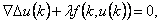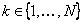,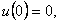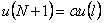where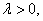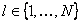, and f is allowed to change sign. By constructing available operators, we shall apply the method of lower solution and the method of topology degree to obtain positive solution of the above problem for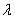on a suitable interval. The associated Green’s function is first given.

1: Paper Source PDF document

Paper's Title:

Refinement Inequalities Among Symmetric Divergence Measures

Author(s):

Inder Jeet Taneja

Departamento de Matemática,
Universidade Federal de Santa Catarina, 88.040-900
Florianópolis, Sc, Brazil

URL: http://www.mtm.ufsc.br/~taneja

Abstract:

There are three classical divergence measures in the literature on information theory and statistics, namely, Jeffryes-Kullback-Leiber’s J-divergence, Sibson-Burbea-Rao’s Jensen- Shannon divegernce and Taneja’s arithemtic - geometric mean divergence. These bear an interesting relationship among each other and are based on logarithmic expressions. The divergence measures like Hellinger discrimination, symmetric χ2divergence, and triangular discrimination are not based on logarithmic expressions. These six divergence measures are symmetric with respect to probability distributions. In this paper some interesting inequalities among these symmetric divergence measures are studied. Refinements of these inequalities are also given. Some inequalities due to Dragomir et al.  are also improved.

1: Paper Source PDF document

Paper's Title:

A general theory of decision making

Author(s):

Frank Hansen

Department of Economics,
University of Copenhagen,
Studiestraede 6, DK-1455 Copenhagen K
Denmark Frank.Hansen@econ.ku.dk
URL: http://www.econ.ku.dk/okofh

Abstract:

We formulate a general theory of decision making based on a lattice of observable events, and we exhibit a large class of representations called the general model. Some of the representations are equivalent to the so called standard model in which observable events are modelled by an algebra of measurable subsets of a state space, while others are not compatible with such a description. We show that the general model collapses to the standard model, if and only if an additional axiom is satisfied. We argue that this axiom is not very natural and thus assert that the standard model may not be general enough to model all relevant phenomena in economics. Using the general model we are (as opposed to Schmeidler ) able to rationalize Ellsberg's paradox without the introduction of non-additive measures.

1: Paper Source PDF document

Paper's Title:

Two Mappings Related to Steffensen's Inequalities

Author(s):

Liang-Cheng Wang

School of Mathematical Science,
Chongqing Institute of Technology,
Xingsheng Lu 4,
Yangjiaping 400050, Chongqing City,
China.
wangliangcheng@163.com

Abstract:

In this paper, we define two mappings closely connected with Steffensen's inequalities, investigate their main properties, give some refinements for Steffensen's inequalities and obtain new inequalities.

1: Paper Source PDF document

Paper's Title:

On Perturbed Reflection Coefficients

Author(s):

J. L. Díaz-Barrero and J. J. Egozcue

Applied Mathematics III,
Barcelona, Spain
jose.luis.diaz@upc.edu
juan.jose.egozcue@upc.edu

Abstract:

Many control and signal processing applications require testing stability of polynomials. Classical tests for locating zeros of polynomials are recursive, but they must be stopped whenever the so called "singular polynomials" appear. These ``singular cases'' are often avoided by perturbing the "singular polynomial". Perturbation techniques although always successful are not proven to be well-founded. Our aim is to give a mathematical foundation to a perturbation method in order to overcome "singular cases" when using Levinson recursion as a testing method. The non-singular polynomials are proven to be dense in the set of all polynomials respect the L˛-norm on the unit circle . The proof is constructive and can be used algorithmically.

1: Paper Source PDF document

Paper's Title:

A New Hardy-Hilbert's Type Inequality for Double Series and its Applications

Author(s):

Mingzhe Gao

Department of Mathematics and Computer Science, Normal College Jishou University,
Jishou Hunan, 416000,
People's Republic of China
mingzhegao1940@yahoo.com.cn

Abstract:

In this paper, it is shown that a new Hardy-Hilbert’s type inequality for double series can be established by introducing a parameter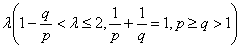and the weight function of the form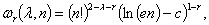where c is Euler constant and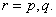And the coefficient is proved to be the best possible. And as the mathematics aesthetics, several important constants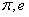and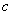appear simultaneously in the coefficient and the weight function when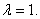In particular, for case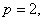some new Hilbert’s type inequalities are obtained. As applications, some extensions of Hardy-Littlewood’s inequality are given.

1: Paper Source PDF document

Paper's Title:

Reconstruction of Discontinuities of Functions Given Noisy Data

Author(s):

Eric D. Mbakop

67A Beaver Park Rd,
Framingham, MA, 01702,
U. S. A.
ericsteve86@yahoo.fr

Abstract:

Suppose one is given noisy data of a discontinuous piecewise-smooth function along with a bound on its second derivative. The locations of the points of discontinuity of f and their jump sizes are not assumed known, but are instead retrieved stably from the noisy data. The novelty of this paper is a numerical method that allows one to locate some of these points of discontinuity with an accuracy that can be made arbitrarily small.

1: Paper Source PDF document

Paper's Title:

Generalizations of Hermite-Hadamard's Inequalities for Log-Convex Functions

Author(s):

Ai-Jun Li

School of Mathematics and Informatics, Henan Polytechnic University,
Jiaozuo City, Henan Province,
454010, China.
liaijun72@163.com

Abstract:

In this article, Hermite-Hadamard's inequalities are extended in terms of the weighted power mean and log-convex function. Several refinements, generalizations and related inequalities are obtained.

1: Paper Source PDF document

Paper's Title:

Existence of Solutions for Third Order Nonlinear Boundary Value Problems

Author(s):

Yue Hu and Zuodong Yang

School of Mathematics and Computer Science, Nanjing Normal University, Jiangsu Nanjing 210097,
China.
huu3y2@163.com

College of Zhongbei, Nanjing Normal University, Jiangsu Nanjing 210046,
China.
zdyang_jin@263.net
yangzuodong@njnu.edu.cn

Abstract:

In this paper, the existence of solution for a class of third order quasilinear ordinary differential equations with nonlinear boundary value problems

p(u"))'=f(t,u,u',u"), u(0)=A, u'(0)=B, R(u'(1),u"(1))=0

is established. The results are obtained by using upper and lower solution methods.

1: Paper Source PDF document

Paper's Title:

Isoperimetric Inequalities for Dual Harmonic Quermassintegrals

Author(s):

Yuan Jun, Zao Lingzhi and Duan Xibo

School of Mathematics and Computer Science,
Nanjing Normal University, Nanjing, 210097,
China.
yuanjun_math@126.com

Department of Mathematics, Nanjing Xiaozhuang University,
Nanjing, 211171,
China.
lzhzhao@163.com

Department of Mathematics, Shandong Water Polytechnic,
Shandong, 276826,
China
dxb1111@sohu.com

Abstract:

In this paper, some isoperimetric inequalities for the dual harmonic quermassintegrals are established.

1: Paper Source PDF document

Paper's Title:

Positive Solutions for Systems of Three-point Nonlinear Boundary Value Problems

Author(s):

J. Henderson and S. K. Ntouyas

Department of Mathematics, Baylor University
Waco, Texas
76798-7328 USA.
Johnny_Henderson@baylor.edu
URL: http://www3.baylor.edu/~Johnny_Henderson

Department of Mathematics, University of Ioannina
451 10 Ioannina,
Greece.
sntouyas@cc.uoi.gr
URL: http://www.math.uoi.gr/~sntouyas

Abstract:

Values of λ are determined for which there exist positive solutions of the system of three-point boundary value problems, u''(t)+ λa(t)f(v(t))=0, v''(t)+λb(t)g(u(t))=0, for 0 < t <1, and satisfying, u(0) = 0, u(1)=α u(η), v(0) = 0, v(1)=α v(η). A Guo-Krasnosel'skii fixed point theorem is applied.

1: Paper Source PDF document

Paper's Title:

The Bernoulli Inequality in Uniformly Complete f-algebras with Identity

Author(s):

Adel Toumi and Mohamed Ali Toumi

Laboratoire de Physique des Liquides Critiques, Département de Physique, Faculté des Sciences de Bizerte, 7021, Zarzouna, Bizerte, TUNISIA

Département de Mathématiques, Faculté des Sciences de Bizerte, 7021, Zarzouna, Bizerte, TUNISIA
MohamedAli.Toumi@fsb.rnu.tn

Abstract:

The main purpose of this paper is to establish with a constructive proof the Bernoulli inequality: let A be a uniformly complete f-algebra with e as unit element, let 1<p<∞, then

(e+a)p≥e+pa

for all a∈A+. As an application we prove the Hölder inequality for positive linear functionals on a uniformly complete f-algebra with identity by using the Minkowski inequality.

1: Paper Source PDF document

Paper's Title:

Some Properties of the Solution of a Second Order Elliptic Abstract Differential Equation

Author(s):

A. Aibeche and K. Laidoune

Mathematics Department, Faculty of Sciences, University Ferhat Abbas, Setif,
Route de Scipion, 19000, Setif,
Algeria
aibeche@univ-setif.dz

Abstract:

In this paper we study a class of non regular boundary value problems for elliptic differential-operator equation of second order with an operator in boundary conditions. We give conditions which guarantee the coerciveness of the solution of the considered problem, the completeness of system of root vectors in Banach-valued functions spaces and we establish the Abel basis property of this system in Hilbert spaces. Finally, we apply this abstract results to a partial differential equation in cylindrical domain.

1: Paper Source PDF document

Paper's Title:

Hyperbolic Barycentric Coordinates

Author(s):

Abraham A. Ungar

Department of Mathematics, North Dakota State University,
Fargo, ND 58105,
USA
Abraham.Ungar@ndsu.edu
URL
: http://math.ndsu.nodak.edu/faculty/ungar/

Abstract:

A powerful and novel way to study Einstein's special theory of relativity and its underlying geometry, the hyperbolic geometry of Bolyai and Lobachevsky, by analogies with classical mechanics and its underlying Euclidean geometry is demonstrated. The demonstration sets the stage for the extension of the notion of barycentric coordinates in Euclidean geometry, first conceived by Möbius in 1827, into hyperbolic geometry. As an example for the application of hyperbolic barycentric coordinates, the hyperbolic midpoint of any hyperbolic segment, and the centroid and orthocenter of any hyperbolic triangle are determined.

1: Paper Source PDF document

Paper's Title:

On the product of M-measures in l-groups

Author(s):

A. Boccuto, B. Riěcan, and A. R. Sambucini

Dipartimento di Matematica e Informatica,
via Vanvitelli, 1 I-06123 Perugia,
Italy.
boccuto@dipmat.unipg.it
URL: http://www.dipmat.unipg.it/~boccuto

Katedra Matematiky, Fakulta Prírodných Vied,
Univerzita Mateja Bela,
Tajovského, 40, Sk-97401 Banská Bystrica,
Slovakia.
riecan@fpv.umb.sk

Dipartimento di Matematica e Informatica,
via Vanvitelli, 1 I-06123 Perugia,
Italy.
matears1@unipg.it
URL: http://www.unipg.it/~matears1

Abstract:

Some extension-type theorems and compactness properties for the
product of l-group-valued M-measures are proved.

1: Paper Source PDF document

Paper's Title:

The Riemann-Stieltjes Integral on Time Scales

Author(s):

D. Mozyrska, E. Pawłuszewicz, D. Torres

Faculty Of Computer Science,
Białystok University Of Technology,
15-351 Białystok,
Poland
d.mozyrska@pb.edu.pl

Department Of Mathematics,
University Of Aveiro,
3810-193 Aveiro,
Portugal
ewa@ua.pt

Department Of Mathematics,
University Of Aveiro,
3810-193 Aveiro,
Portugal
delfim@ua.pt

Abstract:

We study the process of integration on time scales in the sense of Riemann-Stieltjes. Analogues of the classical properties are proved for a generic time scale, and examples are given

1: Paper Source PDF document

Paper's Title:

Generalized Efficient Solutions to One Class of Vector Optimization Problems in Banach Space

Author(s):

Peter I. Kogut, Rosanna Manzo, and Igor V. Nechay

Department of Differential Equations,
Dnipropetrovsk National University,
Naukova str., 13, 49050 Dnipropetrovsk,
Ukraine
p.kogut@i.ua

Dipartimento di Ingegneria Dell’informazione e Matematica Applicata,
Universitŕ di Salerno,
Via Ponte Don Melillo, 84084 Fisciano (Sa),
Italy
manzo@diima.unisa.it

Department of Technical Cybernetics,
Dnipropetrovsk Technical University,
Dnipro
petrovsk,
Ukraine
i.nechay@i.ua

Abstract:

In this paper, we study vector optimization problems in Banach spaces for essentially nonlinear operator equations with additional control and state constraints. We assume that an objective mapping possesses a weakened property of lower semicontinuity and make no assumptions on the interior of the ordering cone. Using the penalization approach we derive both sufficient and necessary conditions for the existence of efficient solutions of the above problems. We also prove the existence of the so-called generalized efficient solutions via the scalarization of some penalized vector optimization problem.

1: Paper Source PDF document

Paper's Title:

Nontrivial Solutions of Singular Superlinear Three-point Boundary Value Problems at Resonance

Author(s):

Feng Wang, Fang Zhang

School of Mathematics and Physics,
Changzou University,
Changzhou, 213164,
China.
fengwang188@163.com

Abstract:

The singular superlinear second order three-point boundary value problems at resonance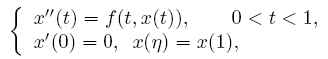are considered under some conditions concerning the first eigenvalues corresponding to the relevant linear operators, where n ∈ (0,1) is a constant, f is allowed to be singular at both t=0 and t=1. The existence results of nontrivial solutions are given by means of the topological degree theory.

1: Paper Source PDF document

Paper's Title:

Shape Diagrams for 2D Compact Sets - Part I: Analytic Convex Sets.

Author(s):

S. Rivollier, J. Debayle and J.-C. Pinoli

Ecole Nationale Supérieure des Mines de Saint-Etienne,

CIS - LPMG, UMR CNRS 5148, 158 cours Fauriel,

42023 Saint-Etienne Cedex 2, France.

Abstract:

Shape diagrams are representations in the Euclidean plane introduced to study 3-dimensional and 2-dimensional compact convex sets. Such a set is represented by a point within a shape diagram whose coordinates are morphometrical functionals defined as normalized ratios of geometrical functionals. Classically, the geometrical functionals are the area, the perimeter, the radii of the inscribed and circumscribed circles, and the minimum and maximum Feret diameters. They allow thirty-one shape diagrams to be built. Most of these shape diagrams can also been applied to more general compact sets than compact convex sets. Starting from these six classical geometrical functionals, a detailed comparative study has been performed in order to analyze the representation relevance and discrimination power of these thirty-one shape diagrams. The purpose of this paper is to present the first part of this study, by focusing on analytic compact convex sets. A set will be called analytic if its boundary is piecewise defined by explicit functions in such a way that the six geometrical functionals can be straightforwardly calculated. The second and third part of the comparative study are published in two following papers [19.20]. They are focused on analytic simply connected sets and convexity discrimination for analytic and discretized simply connected sets, respectively.

1: Paper Source PDF document

Paper's Title:

Shape Diagrams for 2D Compact Sets - Part II: Analytic Simply Connected Sets.

Author(s):

S. Rivollier, J. Debayle and J.-C. Pinoli

Ecole Nationale Supérieure des Mines de Saint-Etienne,

CIS - LPMG, UMR CNRS 5148, 158 cours Fauriel,

42023 Saint-Etienne Cedex 2, France.

Abstract:

Shape diagrams are representations in the Euclidean plane introduced to study 3-dimensional and 2-dimensional compact convex sets. However, they can also been applied to more general compact sets than compact convex sets. A compact set is represented by a point within a shape diagram whose coordinates are morphometrical functionals defined as normalized ratios of geometrical functionals. Classically, the geometrical functionals are the area, the perimeter, the radii of the inscribed and circumscribed circles, and the minimum and maximum Feret diameters. They allow twenty-two shape diagrams to be built. Starting from these six classical geometrical functionals, a detailed comparative study has been performed in order to analyze the representation relevance and discrimination power of these twenty-two shape diagrams. The first part of this study is published in a previous paper 16. It focused on analytic compact convex sets. A set will be called analytic if its boundary is piecewise defined by explicit functions in such a way that the six geometrical functionals can be straightforwardly calculated. The purpose of this paper is to present the second part, by focusing on analytic simply connected compact sets. The third part of the comparative study is published in a following paper 17. It is focused on convexity discrimination for analytic and discretized simply connected compact sets.

1: Paper Source PDF document

Paper's Title:

A Generalization of a Trace Inequality for Positive Definite Matrices

Author(s):

E. V. Belmega, M. Jungers, and S. Lasaulce

Université Paris-Sud Xi, SUPELEC,
Laboratoire Des Signaux Et Systčmes,
Gif-Sur-Yvette,
France.

belmega@lss.supelec.fr
http://veronica.belmega.lss.supelec.fr

CNRS, ENSEM, CRAN, Vandoeuvre,
France.

marc.jungers@cran.uhp-nancy.fr
http://perso.ensem.inpl-nancy.fr/Marc.Jungers/

CNRS, SUPELEC, Laboratoire des Signaux et Systčmes,
Gif-Sur-Yvette,
France.

lasaulce@lss.supelec.fr
http://samson.lasaulce.lss.supelec.fr

Abstract:

In this note, we provide a generalization of the trace inequality derived in [Belmega].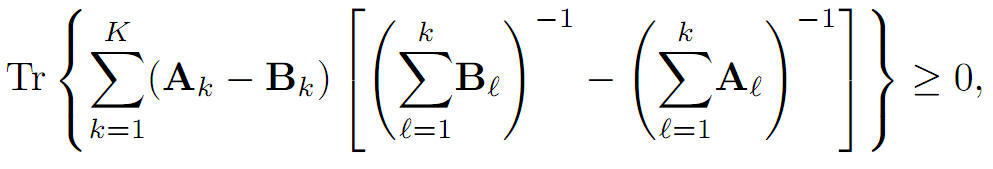More precisely, we prove that for arbitrary K ≥ 1 where Tr(∙) denotes the matrix trace operator, A1, B1 are any positive definite matrices and Ak, Bk for all k∈{2,...,k}, are any positive semidefinite matrices.

1: Paper Source PDF document

Paper's Title:

Necessary and Sufficient Conditions for Cyclic Homogeneous Polynomial Inequalities of Degree Four in Real Variables

Author(s):

Vasile Cirtoaje and Yuanzhe Zhou

Department of Automatic Control and Computers
University of Ploiesti
Romania.
vcirtoaje@upg-ploiesti.ro.

High School Affiliated to Wuhan University, China

Abstract:

In this paper, we give two sets of necessary and sufficient conditions that the inequality f4(x,y,z) ≥ 0 holds for any real numbers x,y,z, where f4(x,y,z) is a cyclic homogeneous polynomial of degree four. In addition, all equality cases of this inequality are analysed. For the particular case in which f4(1,1,1)=0, we get the main result in . Several applications are given to show the effectiveness of the proposed methods.

1: Paper Source PDF document

Paper's Title:

Ap Functions and Maximal Operator

Author(s):

Chunping Xie

Department of Mathematics,
Milwaukee School of Engineering,
Milwaukee, Wisconsin 53202,
U. S. A
.

E-mail: xie@msoe.edu

URL: http://www.msoe.edu/people/chunping.xie

Abstract:

The relationship between Ap functions and Hardy-Littlewood maximal operator on Lp,λ(w), the weighted Morrey space, has been studied. Also the extropolation theorem of Lp,λ(w) has been considered.

1: Paper Source PDF document

Paper's Title:

On the Hyers-Ulam Stability of Homomorphisms and Lie Derivations

Author(s):

Department of Mathematics, Payame Noor University,
P.O. Box: 19395-3697, Tehran,
Iran.

Abstract:

Let A be a Lie Banach*-algebra. For each elements (a, b) and (c, d) in A2:= A * A, by definitions

(a, b) (c, d)= (ac, bd),
|(a, b)|= |a|+ |b|,
(a, b)*= (a*, b*),

A2 can be considered as a Banach*-algebra. This Banach*-algebra is called a Lie Banach*-algebra whenever it is equipped with the following definitions of Lie product: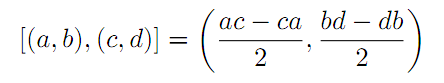for all a, b, c, d in A. Also, if A is a Lie Banach*-algebra, then D: A2→A2 satisfying

D ([ (a, b), (c, d)])= [ D (a, b), (c, d)]+ [(a, b), D (c, d)]

for all \$a, b, c, d∈A, is a Lie derivation on A2. Furthermore, if A is a Lie Banach*-algebra, then D is called a Lie* derivation on A2 whenever D is a Lie derivation with D (a, b)*= D (a*, b*) for all a, b∈A. In this paper, we investigate the Hyers-Ulam stability of Lie Banach*-algebra homomorphisms and Lie* derivations on the Banach*-algebra A2.

1: Paper Source PDF document

Paper's Title:

Hyponormal and K-Quasi-Hyponormal Operators On Semi-Hilbertian Spaces

Author(s):

Ould Ahmed Mahmoud Sid Ahmed and Abdelkader Benali

Mathematics Department,
College of Science,
Aljouf University,
Aljouf 2014,
Saudi Arabia.
E-mail: sididahmed@ju.edu.sa

Mathematics Department, Faculty of Science,
Hassiba Benbouali, University of Chlef,
B.P. 151 Hay Essalem, Chlef 02000,
Algeria.
E-mail: benali4848@gmail.com

Abstract:

Let H be a Hilbert space and let A be a positive bounded operator on H. The semi-inner product < u|v>A:=<Au|v>, u,v H induces a semi-norm || .||A on H. This makes H into a semi-Hilbertian space. In this paper we introduce the notions of hyponormalities and k-quasi-hyponormalities for operators on semi Hilbertian space (H,||.||A), based on the works that studied normal, isometry, unitary and partial isometries operators in these spaces. Also, we generalize some results which are already known for hyponormal and quasi-hyponormal operators. An operator T BA (H) is said to be (A, k)-quasi-hyponormal if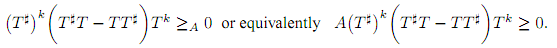1: Paper Source PDF document

Paper's Title:

Credibility Based Fuzzy Entropy Measure

Author(s):

G. Yari, M. Rahimi, B. Moomivand and P. Kumar

Department of Mathematics,
Iran University of Science and Technology,
Tehran,
Iran.
E-mail: Yari@iust.ac.ir
E-mail: Mt_Rahimi@iust.ac.ir
URL: http://www.iust.ac.ir/find.php?item=30.11101.20484.en
URL: http://webpages.iust.ac.ir/mt_rahimi/en.html

Qarzol-hasaneh
Mehr Iran Bank, Tehran,
Iran.
E-mail: B.moomivand@qmb.ir

Department of Mathematics and Statistics,
University of Northern British Columbia,
Prince George, BC,
E-mail: Pranesh.Kumar@unbc.ca

Abstract:

Fuzzy entropy is the entropy of a fuzzy variable, loosely representing the information of uncertainty. This paper, first examines both previous membership and credibility based entropy measures in fuzzy environment, and then suggests an extended credibility based measure which satisfies mostly in Du Luca and Termini axioms. Furthermore, using credibility and the proposed measure, the relative entropy is defined to measure uncertainty between fuzzy numbers. Finally we provide some properties of this Credibility based fuzzy entropy measure and to clarify, give some examples.

1: Paper Source PDF document

Paper's Title:

A Generalization of Viete's Infinite Product and New Mean Iterations

Author(s):

Ryo Nishimura

Department of Frontier Materials
Nagoya Institute of Technology
Gokiso-cho, Showa-ku, Nagoya
Aichi, 466-8555, Japan.
E-mail: rrnishimura@gmail.com

Abstract:

In this paper, we generalize Viéte's infinite product formula by use of Chebyshev polynomials. Furthermore, the infinite product formula for the lemniscate sine is also generalized. Finally, we obtain new mean iterations by use of these infinite product formulas.

1: Paper Source PDF document

Paper's Title:

New Refinements of Hölder's Inequality

Author(s):

Xiu-Fen Ma

College of Mathematical and Computer,
No.9 of Xuefu Road, Hechuan District 401520,
Chongqing City,
The People's Republic of China.
E-mail: maxiufen86@163.com

Abstract:

In this paper, we define two mappings, investigate their properties, obtain some new refinements of Hölder's inequality.

1: Paper Source PDF document

Paper's Title:

New Exact Taylor's Expansions without the Remainder: Application to Finance

Author(s):

Moawia Alghalith

Economics Dept.,
University of the West Indies,
St Augustine,

E-mail: malghalith@gmail.com

Abstract:

We present new exact Taylor's expansions with fixed coefficients and without the remainder. We apply the method to the portfolio model.

Corrigendum.

1: Paper Source PDF document

Paper's Title:

A kind of Function Series and Its Applications

Author(s):

Yang Tianze

Mechanical Engineering,
Shandong University, Xinglongshan Campus,
Jinan, Shandong,
China.

E-mail: qdyangtianze@163.com

Abstract:

A kind of new function series is obtained in this paper. Their theorems and proofs are shown, and some applications are given. We give the expansion form of general integral and the series expansion form of function and the general expansion form of derivative. Using them in the mathematics,we get some unexpected result.

1: Paper Source PDF document

Paper's Title:

Existence and Estimate of the Solution for the Approximate Stochastic Equation to the Viscous Barotropic Gas

Author(s):

R. Benseghir and A. Benchettah

LANOS Laboratory,
PO Box 12,
Annaba, Algeria.
E-mail: benseghirrym@ymail.com, abenchettah@hotmail.com

Abstract:

A stochastic equation of a viscous barotropic gas is considered. The application of Ito formula to a specific functional in an infinite dimensional space allows us to obtain an estimate which is useful to analyse the behavior of the solution. As it is difficult to exploit this estimate, we study an approximate problem. More precisely, we consider the equation of a barotropic viscous gas in Lagrangian coordinates and we add a diffusion of the density. An estimate of energy is obtained to analyse the behavior of the solution for this approximate problem and Galerkin method is used to prove the existence and uniqueness of the solution.

1: Paper Source PDF document

Paper's Title:

Cubic Alternating Harmonic Number Sums

Author(s):

Anthony Sofo

Victoria University,
College of Engineering and Science,
Melbourne City,
Australia.
E-mail: Anthony.Sofo@vu.edu.au

Abstract:

We develop new closed form representations of sums of cubic alternating harmonic numbers and reciprocal binomial coefficients. We also identify a new integral representation for the ζ (4)  constant.

1: Paper Source PDF document

Paper's Title:

An Existence of the Solution to Neutral Stochastic Functional Differential Equations Under the Holder Condition

Author(s):

Young-Ho Kim

Department of Mathematics,
Changwon National University,
Changwon, Gyeongsangnam-do 51140,
Korea.

E-mail: iyhkim@changwon.ac.kr

Abstract:

In this paper, we show the existence and uniqueness of solution of the neutral stochastic functional differential equations under weakened H\"{o}lder condition, a weakened linear growth condition, and a contractive condition. Furthermore, in order to obtain the existence of a solution to the equation we used the Picard sequence.

1: Paper Source PDF document

Paper's Title:

Some New Mappings Related to Weighted Mean Inequalities

Author(s):

Xiu-Fen Ma

College of Mathematical and Computer,
No.9 of Xuefu Road, Hechuan District 401520,
Chongqing City,
The People's Republic of China.
E-mail: maxiufen86@163.com

Abstract:

In this paper, we define four mappings related to weighted mean inequalities, investigate their properties, and obtain some new refinements of weighted mean inequalities.

1: Paper Source PDF document

Paper's Title:

Global Analysis on Riemannian Manifolds

Author(s):

Louis Omenyi and Michael Uchenna

Department of Mathematics, Computer Science, Statistics and Informatics,
Alex Ekwueme Federal University, Ndufu-Alike,
Nigeria.
E-mail: omenyi.louis@funai.edu.ng, michael.uchenna@funai.edu.ng
URL: http://www.funai.edu.ng

Abstract:

In this paper, an exposition of the central concept of global analysis on a Riemannan manifold is given. We extend the theory of smooth vector fields from open subsets of Euclidean space to Riemannan manifolds. Specifically, we prove that a Riemannian manifold admits a unique solution for a system of ordinary differential equations generated by the flow of smooth tangent vectors. The idea of partial differential equations on Riemannian manifold is highlighted on the unit sphere.

1: Paper Source PDF document

Paper's Title:

Some fixed point results in partial S-metric spaces

Author(s):

M. M. Rezaee, S. Sedghi, A. Mukheimer, K. Abodayeh, and Z. D. Mitrovic

Department of Mathematics, Qaemshahr Branch,
Iran.

Department of Mathematics, Qaemshahr Branch,
Iran.
E-mail: sedghi.gh@qaemiau.ac.ir

Department of Mathematics and General Sciences,
KSA.
E-mail: mukheimer@psu.edu.sa

Department of Mathematics and General Sciences,
KSA.
E-mail: kamal@psu.edu.sa

Nonlinear Analysis Research Group,
Faculty of Mathematics and Statistics,
Ton Duc Thang University, Ho Chi Minh City,
Vietnam.
E-mail: zoran.mitrovic@tdtu.edu.vn

Abstract:

We introduce in this article a new class of generalized metric spaces, called partial S-metric spaces. In addition, we also give some interesting results on fixed points in the partial S-metric spaces and some applications.

1: Paper Source PDF document

Paper's Title:

Weyl's theorem for class Q and k - quasi class Q Operators

Author(s):

S. Parvatham and D. Senthilkumar

Department of Mathematics and Humanities,
Sri Ramakrishna Institute of Technology, Coimbatore-10, Tamilnadu,
India.
E-mail: parvathasathish@gmail.com

Post Graduate and Research Department of Mathematics,
India.
E-mail: senthilsenkumhari@gmail.com

Abstract:

In this paper, we give some properties of class  Q  operators. It is proved that every class  Q  operators satisfies Weyl's theorem under the condition that  T2  is isometry. Also we proved that every  k  quasi class  Q  operators is Polaroid and the spectral mapping theorem holds for this class of operator. It will be proved that single valued extension property, Weyl and generalized Weyl's theorem holds for every  k  quasi class  Q  operators.

1: Paper Source PDF document

Paper's Title:

Sharp Inequalities Between Hölder and Stolarsky Means of Two Positive Numbers

Author(s):

M. Bustos Gonzalez and A. I. Stan

The University of Iowa,
Department of Mathematics,
14 MacLean Hall,
Iowa City, Iowa,
USA.
E-mail: margarita-bustosgonzalez@uiowa.edu

The Ohio State University at Marion,
Department of Mathematics,
1465 Mount Vernon Avenue,
Marion, Ohio,
USA.
E-mail: stan.7@osu.edu

Abstract:

Given any index of the Stolarsky means, we find the greatest and least indexes of the H\"older means, such that for any two positive numbers, the Stolarsky mean with the given index is bounded from below and above by the Hölder means with those indexes, of the two positive numbers. Finally, we present a geometric application of this inequality involving the Fermat-Torricelli point of a triangle.

1: Paper Source PDF document

Paper's Title:

Lie Group Theoretic Approach of One-Dimensional Black-Scholes Equation

Author(s):

P. L. Zondi and M. B. Matadi

Department of Mathematical sciences,
Faculty of Sciences & Agriculture, University of Zululand,
P Bag X1001, Kwa-Dlangezwa 3886,
South Africa.
zondip@unizulu.ac.za

Abstract:

This study discusses the Lie Symmetry Analysis of Black-Scholes equation via a modified local one-parameter transformations. It can be argued that the transformation of the Black-Scholes equation is firstly obtained by means of riskless rate. Thereafter, the corresponding determining equations to the reduced equation are found. Furthermore, new symmetries of the Black-Scholes equation are constructed and lead to invariant solutions.

1: Paper Source PDF document

Paper's Title:

A Caratheodory's Approximate Solutions of Stochastic Differential Equations Under the Hölder Condition

Author(s):

Bo-Kyeong Kim and Young-Ho Kim

Department of Mathematics,
Changwon National University,
Changwon, Gyeongsangnam-do 51140,
Korea.
E-mail: claire9576@naver.com
yhkim@changwon.ac.kr

Abstract:

In this paper, based on the theorem of the uniqueness of the solution of the stochastic differential equation, the convergence possibility of the Caratheodory's approximate solution was studied by approximating the unique solution. To obtain this convergence theorem, we used a Hölder condition and a weakened linear growth condition. Furthermore, The auxiliary theorems for the existence and continuity of the Caratheodory's approximate solution were investigated as a prerequisite.

1: Paper Source PDF document

Paper's Title:

A Self Adaptive Method for Solving Split Bilevel Variational Inequalities Problem in Hilbert Spaces

Author(s):

Francis Akutsah1, Ojen Kumar Narain2, Funmilayo Abibat Kasali3 Olawale Kazeem Oyewole4 and Akindele Adebayo Mebawondu5

1School of Mathematics,
Statistics and Computer Science,
University of KwaZulu-Natal, Durban,
South Africa.
E-mail: 216040405@stu.ukzn.ac.za, akutsah@gmail.com

2School of Mathematics,
Statistics and Computer Science,
University of KwaZulu-Natal, Durban,
South Africa.
E-mail: naraino@ukzn.ac.za

3Mountain Top University,
Prayer City, Ogun State,
Nigeria.
E-mail: fkasali@mtu.edu.ng

4Technion-Israel Institute of Technology.
E-mail: 217079141@stu.ukzn.ac.za, oyewoleolawalekazeem@gmail.co

5School of Mathematics,
Statistics and Computer Science,
University of KwaZulu-Natal, Durban,
South Africa.
DST-NRF Centre of Excellence in Mathematical and Statistical Sciences (CoE-MaSS),
Johannesburg,
South Africa.
Mountain Top University,
Prayer City, Ogun State,
Nigeria.
E-mail: dele@aims.ac.za

Abstract:

In this work, we study the split bilevel variational inequality problem in two real Hilbert spaces. We propose a new modified inertial projection and contraction method for solving the aforementioned problem when one of the operators is pseudomonotone and Lipschitz continuous while the other operator is α-strongly monotone. The use of the weakly sequential continuity condition on the Pseudomonotone operator is removed in this work. A Strong convergence theorem of the proposed method is proved under some mild conditions. In addition, some numerical experiments are presented to show the efficiency and implementation of our method in comparison with other methods in the literature in the framework of infinite dimensional Hilbert spaces. The results obtained in this paper extend, generalize and improve several.

1: Paper Source PDF document

Paper's Title:

Topological Aspects of Discrete Switch Dynamical Systems

Author(s):

Faiz Imam and Sharan Gopal

Department of Mathematics,
India.
E-mail: mefaizy@gmail.com

Department of Mathematics,